# 1.6 Add and subtract fractions  (Page 3/4)

 Page 3 / 4

Simplify: $\frac{3a}{4}-\phantom{\rule{0.2em}{0ex}}\frac{8}{9}$ $\frac{3a}{4}·\frac{8}{9}.$

$\frac{27a-32}{36}$ $\frac{2a}{3}$

Simplify: $\frac{4k}{5}-\phantom{\rule{0.2em}{0ex}}\frac{1}{6}$ $\frac{4k}{5}·\frac{1}{6}.$

$\frac{24k-5}{30}$ $\frac{2k}{15}$

## Use the order of operations to simplify complex fractions

We have seen that a complex fraction is a fraction in which the numerator or denominator contains a fraction. The fraction bar indicates division . We simplified the complex fraction $\frac{\frac{3}{4}}{\frac{5}{8}}$ by dividing $\frac{3}{4}$ by $\frac{5}{8}.$

Now we’ll look at complex fractions where the numerator or denominator contains an expression that can be simplified. So we first must completely simplify the numerator and denominator separately using the order of operations. Then we divide the numerator by the denominator.

## How to simplify complex fractions

Simplify: $\frac{{\left(\frac{1}{2}\right)}^{2}}{4+{3}^{2}}.$

## Solution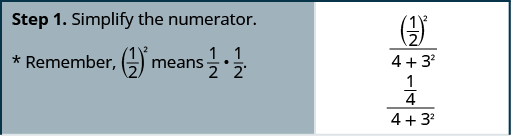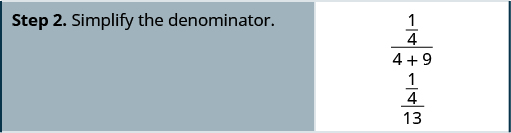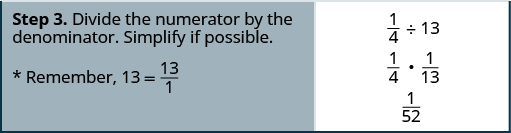Simplify: $\frac{{\left(\frac{1}{3}\right)}^{2}}{{2}^{3}+2}.$

$\frac{1}{90}$

Simplify: $\frac{1+{4}^{2}}{{\left(\frac{1}{4}\right)}^{2}}.$

$272$

## Simplify complex fractions.

1. Simplify the numerator.
2. Simplify the denominator.
3. Divide the numerator by the denominator. Simplify if possible.

Simplify: $\frac{\frac{1}{2}+\frac{2}{3}}{\frac{3}{4}-\phantom{\rule{0.2em}{0ex}}\frac{1}{6}}.$

## Solution

It may help to put parentheses around the numerator and the denominator.

$\begin{array}{cccccc}& & & & & \frac{\left(\frac{1}{2}+\frac{2}{3}\right)}{\left(\frac{3}{4}-\phantom{\rule{0.2em}{0ex}}\frac{1}{6}\right)}\hfill \\ \\ \\ \begin{array}{c}\text{Simplify the numerator (LCD = 6)}\hfill \\ \text{and simplify the denominator (LCD = 12).}\hfill \end{array}\hfill & & & & & \hfill \frac{\left(\frac{3}{6}+\frac{4}{6}\right)}{\left(\frac{9}{12}-\phantom{\rule{0.2em}{0ex}}\frac{2}{12}\right)}\hfill \\ \\ \\ \text{Simplify.}\hfill & & & & & \hfill \frac{\left(\frac{7}{6}\right)}{\left(\frac{7}{12}\right)}\hfill \\ \\ \\ \text{Divide the numerator by the denominator.}\hfill & & & & & \hfill \frac{7}{6}÷\frac{7}{12}\hfill \\ \\ \\ \text{Simplify.}\hfill & & & & & \hfill \frac{7}{6}·\frac{12}{7}\hfill \\ \\ \\ \text{Divide out common factors.}\hfill & & & & & \hfill \frac{7·6·2}{6·7}\hfill \\ \\ \\ \text{Simplify.}\hfill & & & & & \hfill 2\hfill \end{array}$

Simplify: $\frac{\frac{1}{3}+\frac{1}{2}}{\frac{3}{4}-\phantom{\rule{0.2em}{0ex}}\frac{1}{3}}.$

2

Simplify: $\frac{\frac{2}{3}-\phantom{\rule{0.2em}{0ex}}\frac{1}{2}}{\frac{1}{4}+\frac{1}{3}}.$

$\frac{2}{7}$

## Evaluate variable expressions with fractions

We have evaluated expressions before, but now we can evaluate expressions with fractions. Remember, to evaluate an expression, we substitute the value of the variable into the expression and then simplify.

Evaluate $x+\frac{1}{3}$ when $x=-\phantom{\rule{0.2em}{0ex}}\frac{1}{3}$ $x=-\phantom{\rule{0.2em}{0ex}}\frac{3}{4}.$

1. To evaluate $x+\frac{1}{3}$ when $x=-\phantom{\rule{0.2em}{0ex}}\frac{1}{3},$ substitute $-\phantom{\rule{0.2em}{0ex}}\frac{1}{3}$ for $x$ in the expression.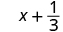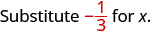Simplify. $\phantom{\rule{18em}{0ex}}$ 0

2. To evaluate $x+\frac{1}{3}$ when $x=-\phantom{\rule{0.2em}{0ex}}\frac{3}{4},$ we substitute $-\phantom{\rule{0.2em}{0ex}}\frac{3}{4}$ for x in the expression.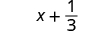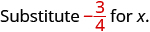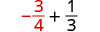Rewrite as equivalent fractions with the LCD, 12.Simplify.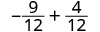Add. $-\phantom{\rule{0.2em}{0ex}}\frac{5}{12}$

Evaluate $x+\frac{3}{4}$ when $x=-\phantom{\rule{0.2em}{0ex}}\frac{7}{4}$ $x=-\phantom{\rule{0.2em}{0ex}}\frac{5}{4}.$

$-1$ $-\phantom{\rule{0.2em}{0ex}}\frac{1}{2}$

Evaluate $y+\frac{1}{2}$ when $y=\frac{2}{3}$ $y=-\phantom{\rule{0.2em}{0ex}}\frac{3}{4}.$

$\frac{7}{6}$ $-\phantom{\rule{0.2em}{0ex}}\frac{1}{12}$

Evaluate $-\phantom{\rule{0.2em}{0ex}}\frac{5}{6}-y$ when $y=-\phantom{\rule{0.2em}{0ex}}\frac{2}{3}.$

## Solution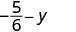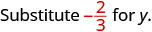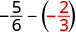Rewrite as equivalent fractions with the LCD, 6.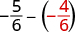Subtract.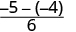Simplify. $-\phantom{\rule{0.2em}{0ex}}\frac{1}{6}$

Evaluate $-\phantom{\rule{0.2em}{0ex}}\frac{1}{2}-y$ when $y=-\phantom{\rule{0.2em}{0ex}}\frac{1}{4}.$

$-\phantom{\rule{0.2em}{0ex}}\frac{1}{4}$

Evaluate $-\phantom{\rule{0.2em}{0ex}}\frac{3}{8}-y$ when $x=-\phantom{\rule{0.2em}{0ex}}\frac{5}{2}.$

$-\phantom{\rule{0.2em}{0ex}}\frac{17}{8}$

Evaluate $2{x}^{2}y$ when $x=\frac{1}{4}$ and $y=-\phantom{\rule{0.2em}{0ex}}\frac{2}{3}.$

## Solution

Substitute the values into the expression.

 $2{x}^{2}y$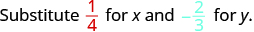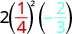Simplify exponents first. $2\left(\frac{1}{16}\right)\left(-\phantom{\rule{0.2em}{0ex}}\frac{2}{3}\right)$ Multiply. Divide out the common factors. Notice we write 16 as $2\cdot 2\cdot 4$ to make it easy to remove common factors. $-\phantom{\rule{0.2em}{0ex}}\frac{\overline{)2}\cdot 1\cdot \overline{)2}}{\overline{)2}\cdot \overline{)2}\cdot 4\cdot 3}$ Simplify. $-\phantom{\rule{0.2em}{0ex}}\frac{1}{12}$

Evaluate $3a{b}^{2}$ when $a=-\phantom{\rule{0.2em}{0ex}}\frac{2}{3}$ and $b=-\phantom{\rule{0.2em}{0ex}}\frac{1}{2}.$

$-\phantom{\rule{0.2em}{0ex}}\frac{1}{2}$

Evaluate $4{c}^{3}d$ when $c=-\phantom{\rule{0.2em}{0ex}}\frac{1}{2}$ and $d=-\phantom{\rule{0.2em}{0ex}}\frac{4}{3}.$

$\frac{2}{3}$

The next example will have only variables, no constants.

Evaluate $\frac{p+q}{r}$ when $p=-4,q=-2,\phantom{\rule{0.2em}{0ex}}\text{and}\phantom{\rule{0.2em}{0ex}}r=8.$

## Solution

To evaluate $\frac{p+q}{r}$ when $p=-4,q=-2,\phantom{\rule{0.2em}{0ex}}\text{and}\phantom{\rule{0.2em}{0ex}}r=8,$ we substitute the values into the expression.

 $\frac{p+q}{r}$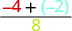Add in the numerator first. $\frac{-6}{8}$ Simplify. $-\phantom{\rule{0.2em}{0ex}}\frac{3}{4}$

Evaluate $\frac{a+b}{c}$ when $a=-8,b=-7,\phantom{\rule{0.2em}{0ex}}\text{and}\phantom{\rule{0.2em}{0ex}}c=6.$

$-\phantom{\rule{0.2em}{0ex}}\frac{5}{2}$

Evaluate $\frac{x+y}{z}$ when $x=9,y=-18,\phantom{\rule{0.2em}{0ex}}\text{and}\phantom{\rule{0.2em}{0ex}}z=-6.$

$\frac{3}{2}$

## Key concepts

• Fraction Addition and Subtraction: If $a,b,\phantom{\rule{0.2em}{0ex}}\text{and}\phantom{\rule{0.2em}{0ex}}c$ are numbers where $c\ne 0,$ then
$\frac{a}{c}+\frac{b}{c}=\frac{a+b}{c}$ and $\frac{a}{c}-\phantom{\rule{0.2em}{0ex}}\frac{b}{c}=\frac{a-b}{c}.$
To add or subtract fractions, add or subtract the numerators and place the result over the common denominator.
• Strategy for Adding or Subtracting Fractions
1. Do they have a common denominator?
Yes—go to step 2.
No—Rewrite each fraction with the LCD (Least Common Denominator). Find the LCD. Change each fraction into an equivalent fraction with the LCD as its denominator.
2. Add or subtract the fractions.
3. Simplify, if possible. To multiply or divide fractions, an LCD IS NOT needed. To add or subtract fractions, an LCD IS needed.
• Simplify Complex Fractions
1. Simplify the numerator.
2. Simplify the denominator.
3. Divide the numerator by the denominator. Simplify if possible.

## Practice makes perfect

Add and Subtract Fractions with a Common Denominator

$\frac{6}{13}+\frac{5}{13}$

$\frac{11}{13}$

$\frac{4}{15}+\frac{7}{15}$

$\frac{x}{4}+\frac{3}{4}$

$\frac{x+3}{4}$

$\frac{8}{q}+\frac{6}{q}$

$-\phantom{\rule{0.2em}{0ex}}\frac{3}{16}+\left(-\phantom{\rule{0.2em}{0ex}}\frac{7}{16}\right)$

$-\phantom{\rule{0.2em}{0ex}}\frac{5}{8}$

$-\phantom{\rule{0.2em}{0ex}}\frac{5}{16}+\left(-\phantom{\rule{0.2em}{0ex}}\frac{9}{16}\right)$

$-\phantom{\rule{0.2em}{0ex}}\frac{8}{17}+\frac{15}{17}$

$\frac{7}{17}$

$-\phantom{\rule{0.2em}{0ex}}\frac{9}{19}+\frac{17}{19}$

$\frac{6}{13}+\left(-\phantom{\rule{0.2em}{0ex}}\frac{10}{13}\right)+\left(-\phantom{\rule{0.2em}{0ex}}\frac{12}{13}\right)$

$-\phantom{\rule{0.2em}{0ex}}\frac{16}{13}$

$\frac{5}{12}+\left(-\phantom{\rule{0.2em}{0ex}}\frac{7}{12}\right)+\left(-\phantom{\rule{0.2em}{0ex}}\frac{11}{12}\right)$

In the following exercises, subtract.

$\frac{11}{15}-\phantom{\rule{0.2em}{0ex}}\frac{7}{15}$

$\frac{4}{15}$

$\frac{9}{13}-\phantom{\rule{0.2em}{0ex}}\frac{4}{13}$

$\frac{11}{12}-\phantom{\rule{0.2em}{0ex}}\frac{5}{12}$

$\frac{1}{2}$

$\frac{7}{12}-\phantom{\rule{0.2em}{0ex}}\frac{5}{12}$

$\frac{19}{21}-\phantom{\rule{0.2em}{0ex}}\frac{4}{21}$

$\frac{5}{7}$

$\frac{17}{21}-\phantom{\rule{0.2em}{0ex}}\frac{8}{21}$

$\frac{5y}{8}-\phantom{\rule{0.2em}{0ex}}\frac{7}{8}$

$\frac{5y-7}{8}$

$\frac{11z}{13}-\phantom{\rule{0.2em}{0ex}}\frac{8}{13}$

$-\phantom{\rule{0.2em}{0ex}}\frac{23}{u}-\phantom{\rule{0.2em}{0ex}}\frac{15}{u}$

$-\phantom{\rule{0.2em}{0ex}}\frac{38}{u}$

$-\phantom{\rule{0.2em}{0ex}}\frac{29}{v}-\phantom{\rule{0.2em}{0ex}}\frac{26}{v}$

$-\phantom{\rule{0.2em}{0ex}}\frac{3}{5}-\left(-\phantom{\rule{0.2em}{0ex}}\frac{4}{5}\right)$

$\frac{1}{5}$

$-\phantom{\rule{0.2em}{0ex}}\frac{3}{7}-\left(-\phantom{\rule{0.2em}{0ex}}\frac{5}{7}\right)$

$-\phantom{\rule{0.2em}{0ex}}\frac{7}{9}-\left(-\phantom{\rule{0.2em}{0ex}}\frac{5}{9}\right)$

$-\phantom{\rule{0.2em}{0ex}}\frac{2}{9}$

$-\phantom{\rule{0.2em}{0ex}}\frac{8}{11}-\left(-\phantom{\rule{0.2em}{0ex}}\frac{5}{11}\right)$

Mixed Practice

In the following exercises, simplify.

$-\phantom{\rule{0.2em}{0ex}}\frac{5}{18}·\frac{9}{10}$

$-\phantom{\rule{0.2em}{0ex}}\frac{1}{4}$

$-\phantom{\rule{0.2em}{0ex}}\frac{3}{14}·\frac{7}{12}$

$\frac{n}{5}-\phantom{\rule{0.2em}{0ex}}\frac{4}{5}$

$\frac{n-4}{5}$

$\frac{6}{11}-\phantom{\rule{0.2em}{0ex}}\frac{s}{11}$

$-\phantom{\rule{0.2em}{0ex}}\frac{7}{24}+\frac{2}{24}$

$-\phantom{\rule{0.2em}{0ex}}\frac{5}{24}$

$-\phantom{\rule{0.2em}{0ex}}\frac{5}{18}+\frac{1}{18}$

$\frac{8}{15}÷\frac{12}{5}$

$\frac{2}{9}$

$\frac{7}{12}÷\frac{9}{28}$

Add or Subtract Fractions with Different Denominators

In the following exercises, add or subtract.

$\frac{1}{2}+\frac{1}{7}$

$\frac{9}{14}$

$\frac{1}{3}+\frac{1}{8}$

$\frac{1}{3}-\left(-\phantom{\rule{0.2em}{0ex}}\frac{1}{9}\right)$

$\frac{4}{9}$

$\frac{1}{4}-\left(-\phantom{\rule{0.2em}{0ex}}\frac{1}{8}\right)$

$\frac{7}{12}+\frac{5}{8}$

$\frac{29}{24}$

$\frac{5}{12}+\frac{3}{8}$

$\frac{7}{12}-\phantom{\rule{0.2em}{0ex}}\frac{9}{16}$

$\frac{1}{48}$

$\frac{7}{16}-\phantom{\rule{0.2em}{0ex}}\frac{5}{12}$

$\frac{2}{3}-\phantom{\rule{0.2em}{0ex}}\frac{3}{8}$

$\frac{7}{24}$

$\frac{5}{6}-\phantom{\rule{0.2em}{0ex}}\frac{3}{4}$

$-\phantom{\rule{0.2em}{0ex}}\frac{11}{30}+\frac{27}{40}$

$\frac{37}{120}$

$-\phantom{\rule{0.2em}{0ex}}\frac{9}{20}+\frac{17}{30}$

$-\phantom{\rule{0.2em}{0ex}}\frac{13}{30}+\frac{25}{42}$

$\frac{17}{105}$

$-\phantom{\rule{0.2em}{0ex}}\frac{23}{30}+\frac{5}{48}$

$-\phantom{\rule{0.2em}{0ex}}\frac{39}{56}-\phantom{\rule{0.2em}{0ex}}\frac{22}{35}$

$-\phantom{\rule{0.2em}{0ex}}\frac{53}{40}$

$-\phantom{\rule{0.2em}{0ex}}\frac{33}{49}-\phantom{\rule{0.2em}{0ex}}\frac{18}{35}$

$-\phantom{\rule{0.2em}{0ex}}\frac{2}{3}-\left(-\phantom{\rule{0.2em}{0ex}}\frac{3}{4}\right)$

$\frac{1}{12}$

$-\phantom{\rule{0.2em}{0ex}}\frac{3}{4}-\left(-\phantom{\rule{0.2em}{0ex}}\frac{4}{5}\right)$

$1+\frac{7}{8}$

$\frac{15}{8}$

$1-\phantom{\rule{0.2em}{0ex}}\frac{3}{10}$

$\frac{x}{3}+\frac{1}{4}$

$\frac{4x+3}{12}$

$\frac{y}{2}+\frac{2}{3}$

$\frac{y}{4}-\phantom{\rule{0.2em}{0ex}}\frac{3}{5}$

$\frac{4y-12}{20}$

$\frac{x}{5}-\phantom{\rule{0.2em}{0ex}}\frac{1}{4}$

Mixed Practice

In the following exercises, simplify.

$\frac{2}{3}+\frac{1}{6}$ $\frac{2}{3}÷\frac{1}{6}$

$\frac{5}{6}$ 4

$-\phantom{\rule{0.2em}{0ex}}\frac{2}{5}-\phantom{\rule{0.2em}{0ex}}\frac{1}{8}$ $-\phantom{\rule{0.2em}{0ex}}\frac{2}{5}·\frac{1}{8}$

$\frac{5n}{6}÷\frac{8}{15}$ $\frac{5n}{6}-\phantom{\rule{0.2em}{0ex}}\frac{8}{15}$

$\frac{25n}{16}$ $\frac{25n-16}{30}$

$\frac{3a}{8}÷\frac{7}{12}$ $\frac{3a}{8}-\phantom{\rule{0.2em}{0ex}}\frac{7}{12}$

$-\phantom{\rule{0.2em}{0ex}}\frac{3}{8}÷\left(-\phantom{\rule{0.2em}{0ex}}\frac{3}{10}\right)$

$\frac{5}{4}$

$-\phantom{\rule{0.2em}{0ex}}\frac{5}{12}÷\left(-\phantom{\rule{0.2em}{0ex}}\frac{5}{9}\right)$

$-\phantom{\rule{0.2em}{0ex}}\frac{3}{8}+\frac{5}{12}$

$\frac{1}{24}$

$-\phantom{\rule{0.2em}{0ex}}\frac{1}{8}+\frac{7}{12}$

$\frac{5}{6}-\phantom{\rule{0.2em}{0ex}}\frac{1}{9}$

$\frac{13}{18}$

$\frac{5}{9}-\phantom{\rule{0.2em}{0ex}}\frac{1}{6}$

$-\phantom{\rule{0.2em}{0ex}}\frac{7}{15}-\phantom{\rule{0.2em}{0ex}}\frac{y}{4}$

$\frac{-28-15y}{60}$

$-\phantom{\rule{0.2em}{0ex}}\frac{3}{8}-\phantom{\rule{0.2em}{0ex}}\frac{x}{11}$

$\frac{11}{12a}·\frac{9a}{16}$

$\frac{33}{64}$

$\frac{10y}{13}·\frac{8}{15y}$

Use the Order of Operations to Simplify Complex Fractions

In the following exercises, simplify.

$\frac{{2}^{3}+{4}^{2}}{{\left(\frac{2}{3}\right)}^{2}}$

54

$\frac{{3}^{3}-{3}^{2}}{{\left(\frac{3}{4}\right)}^{2}}$

$\frac{{\left(\frac{3}{5}\right)}^{2}}{{\left(\frac{3}{7}\right)}^{2}}$

$\frac{49}{25}$

$\frac{{\left(\frac{3}{4}\right)}^{2}}{{\left(\frac{5}{8}\right)}^{2}}$

$\frac{2}{\frac{1}{3}+\frac{1}{5}}$

$\frac{15}{4}$

$\frac{5}{\frac{1}{4}+\frac{1}{3}}$

$\frac{\frac{7}{8}-\phantom{\rule{0.2em}{0ex}}\frac{2}{3}}{\frac{1}{2}+\frac{3}{8}}$

$\frac{5}{21}$

$\frac{\frac{3}{4}-\phantom{\rule{0.2em}{0ex}}\frac{3}{5}}{\frac{1}{4}+\frac{2}{5}}$

$\frac{1}{2}+\frac{2}{3}·\frac{5}{12}$

$\frac{7}{9}$

$\frac{1}{3}+\frac{2}{5}·\frac{3}{4}$

$1-\phantom{\rule{0.2em}{0ex}}\frac{3}{5}÷\frac{1}{10}$

$-5$

$1-\phantom{\rule{0.2em}{0ex}}\frac{5}{6}÷\frac{1}{12}$

$\frac{2}{3}+\frac{1}{6}+\frac{3}{4}$

$\frac{19}{12}$

$\frac{2}{3}+\frac{1}{4}+\frac{3}{5}$

$\frac{3}{8}-\phantom{\rule{0.2em}{0ex}}\frac{1}{6}+\frac{3}{4}$

$\frac{23}{24}$

$\frac{2}{5}+\frac{5}{8}-\phantom{\rule{0.2em}{0ex}}\frac{3}{4}$

$12\left(\frac{9}{20}-\phantom{\rule{0.2em}{0ex}}\frac{4}{15}\right)$

$\frac{11}{5}$

$8\left(\frac{15}{16}-\phantom{\rule{0.2em}{0ex}}\frac{5}{6}\right)$

$\frac{\frac{5}{8}+\frac{1}{6}}{\frac{19}{24}}$

1

$\frac{\frac{1}{6}+\frac{3}{10}}{\frac{14}{30}}$

$\left(\frac{5}{9}+\frac{1}{6}\right)÷\left(\frac{2}{3}-\phantom{\rule{0.2em}{0ex}}\frac{1}{2}\right)$

$\frac{13}{3}$

$\left(\frac{3}{4}+\frac{1}{6}\right)÷\left(\frac{5}{8}-\phantom{\rule{0.2em}{0ex}}\frac{1}{3}\right)$

Evaluate Variable Expressions with Fractions

In the following exercises, evaluate.

$x+\left(-\phantom{\rule{0.2em}{0ex}}\frac{5}{6}\right)$ when
$x=\frac{1}{3}$
$x=-\phantom{\rule{0.2em}{0ex}}\frac{1}{6}$

$-\phantom{\rule{0.2em}{0ex}}\frac{1}{2}$ $-1$

$x+\left(-\phantom{\rule{0.2em}{0ex}}\frac{11}{12}\right)$ when
$x=\frac{11}{12}$
$x=\frac{3}{4}$

$x-\phantom{\rule{0.2em}{0ex}}\frac{2}{5}$ when
$x=\frac{3}{5}$
$x=-\phantom{\rule{0.2em}{0ex}}\frac{3}{5}$

$\frac{1}{5}$ $-1$

$x-\phantom{\rule{0.2em}{0ex}}\frac{1}{3}$ when
$x=\frac{2}{3}$
$x=-\phantom{\rule{0.2em}{0ex}}\frac{2}{3}$

$\frac{7}{10}-w$ when
$w=\frac{1}{2}$
$w=-\phantom{\rule{0.2em}{0ex}}\frac{1}{2}$

$\frac{1}{5}$ $\frac{6}{5}$

$\frac{5}{12}-w$ when
$w=\frac{1}{4}$
$w=-\phantom{\rule{0.2em}{0ex}}\frac{1}{4}$

$2{x}^{2}{y}^{3}$ when $x=-\phantom{\rule{0.2em}{0ex}}\frac{2}{3}$ and $y=-\phantom{\rule{0.2em}{0ex}}\frac{1}{2}$

$-\phantom{\rule{0.2em}{0ex}}\frac{1}{9}$

$8{u}^{2}{v}^{3}$ when $u=-\phantom{\rule{0.2em}{0ex}}\frac{3}{4}$ and $v=-\phantom{\rule{0.2em}{0ex}}\frac{1}{2}$

$\frac{a+b}{a-b}$ when $a=-3,b=8$

$-\phantom{\rule{0.2em}{0ex}}\frac{5}{11}$

$\frac{r-s}{r+s}$ when $r=10,s=-5$

## Everyday math

Decorating Laronda is making covers for the throw pillows on her sofa. For each pillow cover, she needs $\frac{1}{2}$ yard of print fabric and $\frac{3}{8}$ yard of solid fabric. What is the total amount of fabric Laronda needs for each pillow cover?

$\frac{7}{8}$ yard

Baking Vanessa is baking chocolate chip cookies and oatmeal cookies. She needs $\frac{1}{2}$ cup of sugar for the chocolate chip cookies and $\frac{1}{4}$ of sugar for the oatmeal cookies. How much sugar does she need altogether?

## Writing exercises

Why do you need a common denominator to add or subtract fractions? Explain.

How do you find the LCD of 2 fractions?

## Self check

After completing the exercises, use this checklist to evaluate your mastery of the objectives of this section.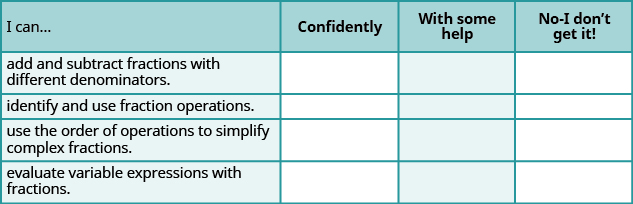After looking at the checklist, do you think you are well-prepared for the next chapter? Why or why not?

Lucinda has a pocketful of dimes and quarters with a value of $6.20. The number of dimes is 18 more than 3 times the number of quarters. How many dimes and how many quarters does Lucinda have? Rhonda Reply Find an equation for the line that passes through the point P ( 0 , − 4 ) and has a slope 8/9 . Gabriel Reply is that a negative 4 or positive 4? Felix y = mx + b Felix if negative -4, then -4=8/9(0) + b Felix -4=b Felix if positive 4, then 4=b Felix then plug in y=8/9x - 4 or y=8/9x+4 Felix Macario is making 12 pounds of nut mixture with macadamia nuts and almonds. macadamia nuts cost$9 per pound and almonds cost $5.25 per pound. how many pounds of macadamia nuts and how many pounds of almonds should macario use for the mixture to cost$6.50 per pound to make?
Nga and Lauren bought a chest at a flea market for $50. They re-finished it and then added a 350 % mark - up Makaila Reply the sum of two Numbers is 19 and their difference is 15 Abdulai Reply 2, 17 Jose interesting saw Felecia left her home to visit her daughter, driving 45mph. Her husband waited for the dog sitter to arrive and left home 20 minutes, or 13 hour later. He drove 55mph to catch up to Felecia. How long before he reaches her? Rafi Reply integer greater than 2 and less than 12 Emily Reply 2 < x < 12 Felix I'm guessing you are doing inequalities... Felix Actually, translating words into algebraic expressions / equations... Felix hi Darianna hello Mister Eric here Eric He charges$125 per job. His monthly expenses are $1,600. How many jobs must he work in order to make a profit of at least$2,400?
at least 20
Ayla
what are the steps?
Alicia
6.4 jobs
Grahame
32
Grahame
1600+2400= total amount with expenses. 4000/125= number of jobs needed to make that min profit of 2400. answer is 32
Orlando
He must work 32 jobs to make a profit
POP
what is algebra
repeated addition and subtraction of the order of operations. i love algebra I'm obsessed.
Shemiah
hi
Krekar
Eric here. I'm a parent. 53 years old. I have never taken algebra. I want to learn.
Eric
One-fourth of the candies in a bag of M&M’s are red. If there are 23 red candies, how many candies are in the bag?
they are 92 candies in the bag
POP
rectangular field solutions
What is this?
Donna
t
muqtaar
the proudact of 3x^3-5×^2+3 and 2x^2+5x-4 in z7[x]/ is
?
Choli
a rock is thrown directly upward with an initial velocity of 96feet per second from a cliff 190 feet above a beach. The hight of tha rock above the beach after t second is given by the equation h=_16t^2+96t+190
Usman
Stella bought a dinette set on sale for $725. The original price was$1,299. To the nearest tenth of a percent, what was the rate of discount?
44.19%
Scott
40.22%
Terence
44.2%
Orlando
I don't know
Donna
if you want the discounted price subtract $725 from$1299. then divide the answer by $1299. you get 0.4419... but as percent you get 44.19... but to the nearest tenth... round .19 to .2 and you get 44.2% Orlando you could also just divide$725/\$1299 and then subtract it from 1. then you get the same answer.
Orlando
p mulripied-5 and add 30 to it
Tausif
Tausif
how
muqtaar
Can you explain further
p mulripied-5 and add to 30
Tausif
-5p+30?
Corey
p=-5+30
JacobBy OpenStaxBy Madison ChristianBy OpenStaxBy Ryan LoweBy Richley CrapoBy Edward BitonBy OpenStaxByBy Madison ChristianBy Robert Murphy